# 线性表详解，map函数用法详解，vector函数用法（近万字详解）

1.简介：

2.实现：

（1）构建节点结构体：

（2）初始化：

（3）添加元素：

（4）去除元素：

3.优缺点：

（1）优点：

（2）缺点：

4.例题：

1.map的简介：

2.map的基本用法：

（1）map的定义：

（2）map的赋值：

（3）判断是否插入成功：

（4）erase操作：

（5）find操作：

（6）clear操作：

（7）size操作：

（8）读取单个value：

（9）遍历读取map中元素：

（10）map与其他STL嵌套：

3.例题：

1.vector简介：

2.vector用法：

（1）开头，结尾表示

（2）清空操作

（3）检验是否为空

（4） 删去最后一个元素

（5）删除操作

（6）中间插入操作

（7）尾部插入操作

（8）元素个数

（9）最大容量

（10）调节元素个数

（11）调节容量大小

## 一.双向链表：

### 1.简介：

链表即将一串数字连接在一起，形成一个闭环，如下图所示：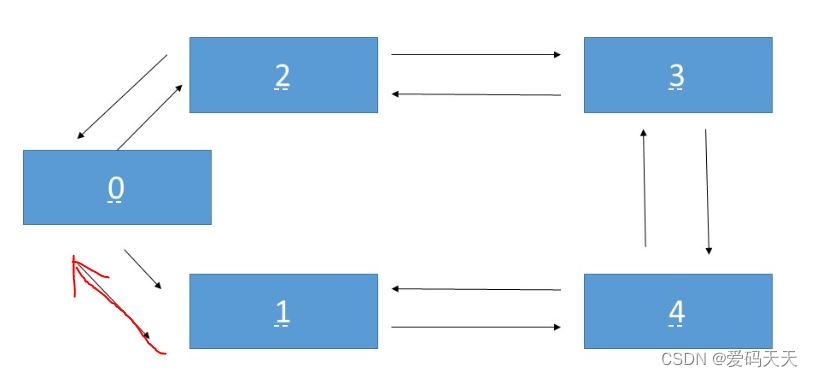（2） 该元素右边的值；

### 2.实现：

#### （1）构建节点结构体：

struct pot
{
int left;
int right;
};


#### （2）初始化：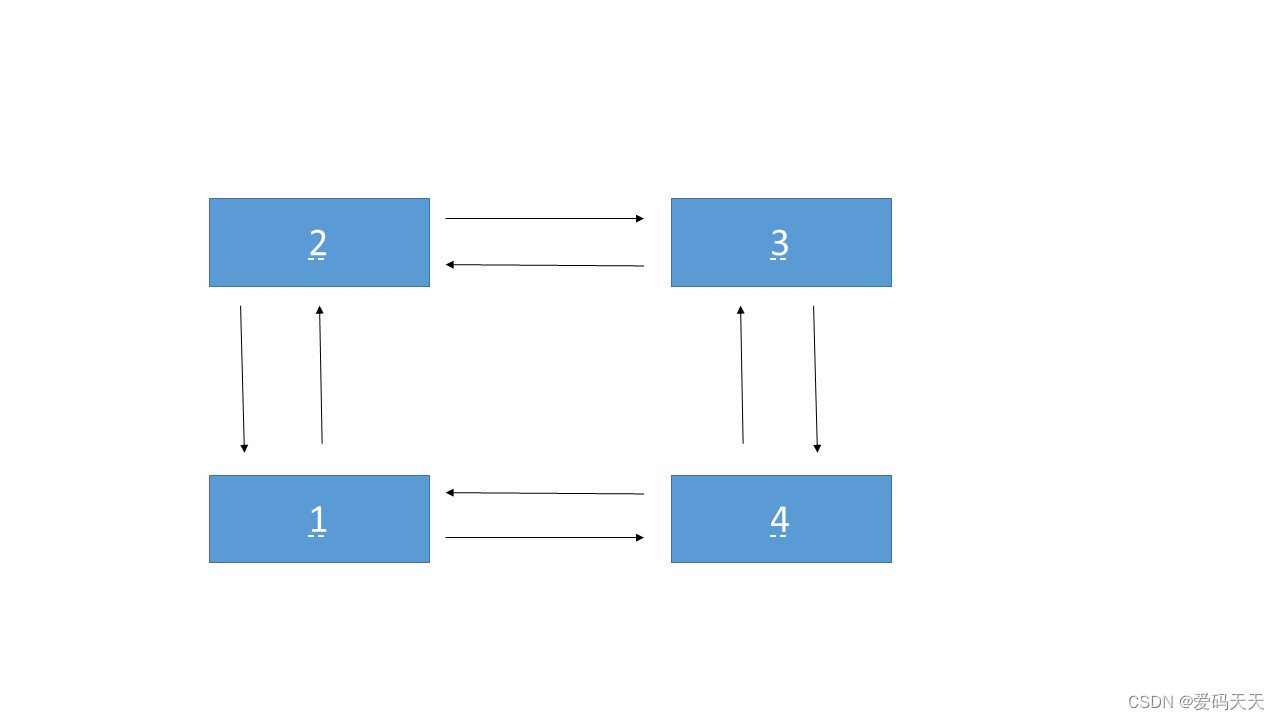struct pot p;
//先开15个结构体
p.left=0;
//等于自身
p.right=0;
//等于自身

相当于0自己连着自己；

#### （3）添加元素：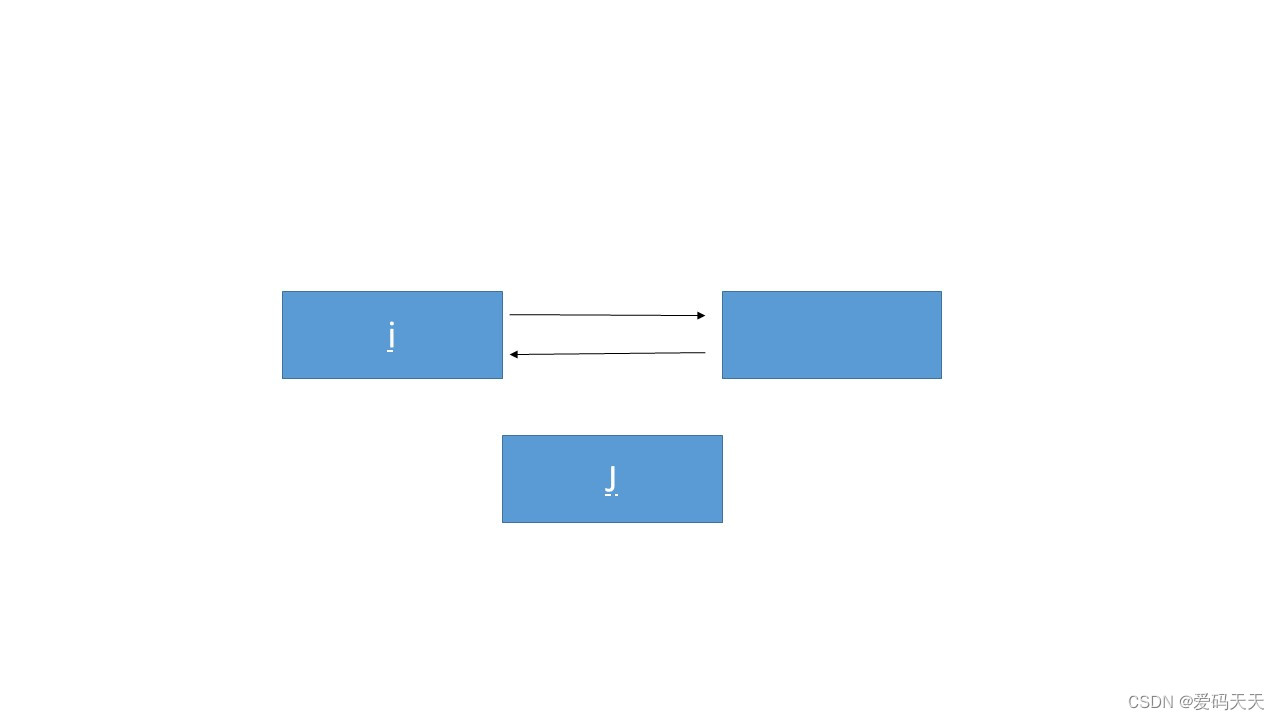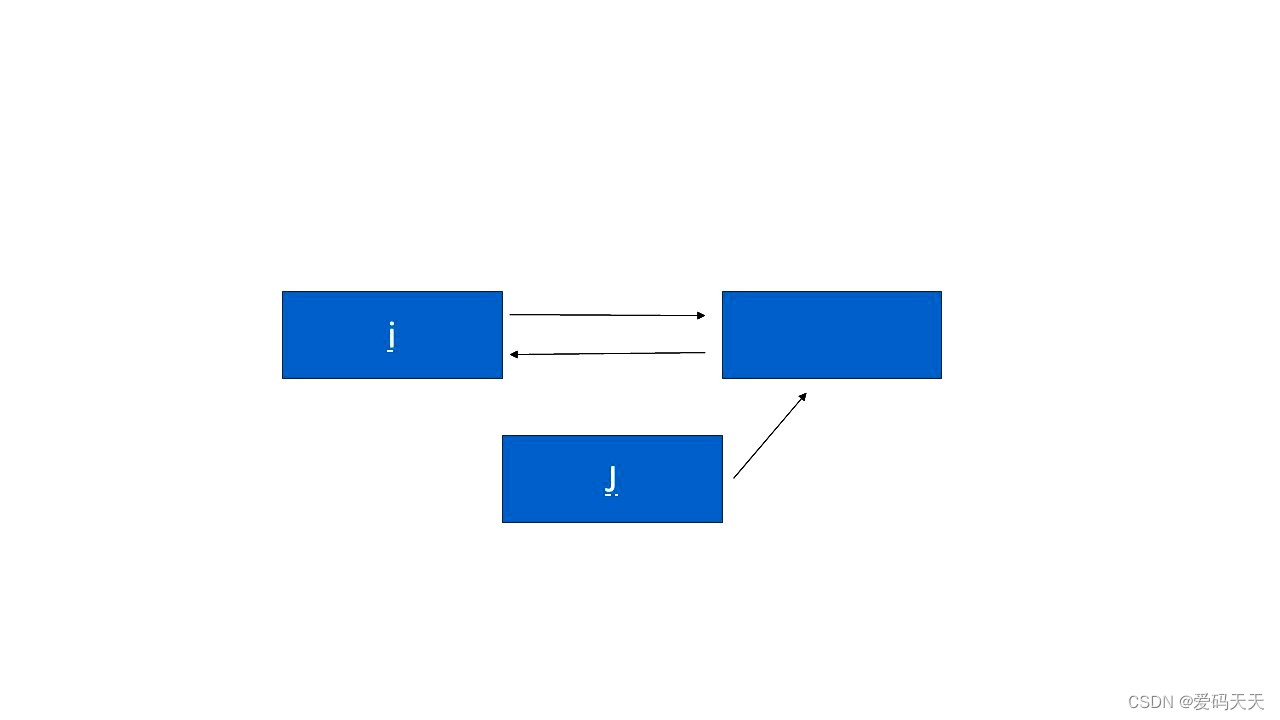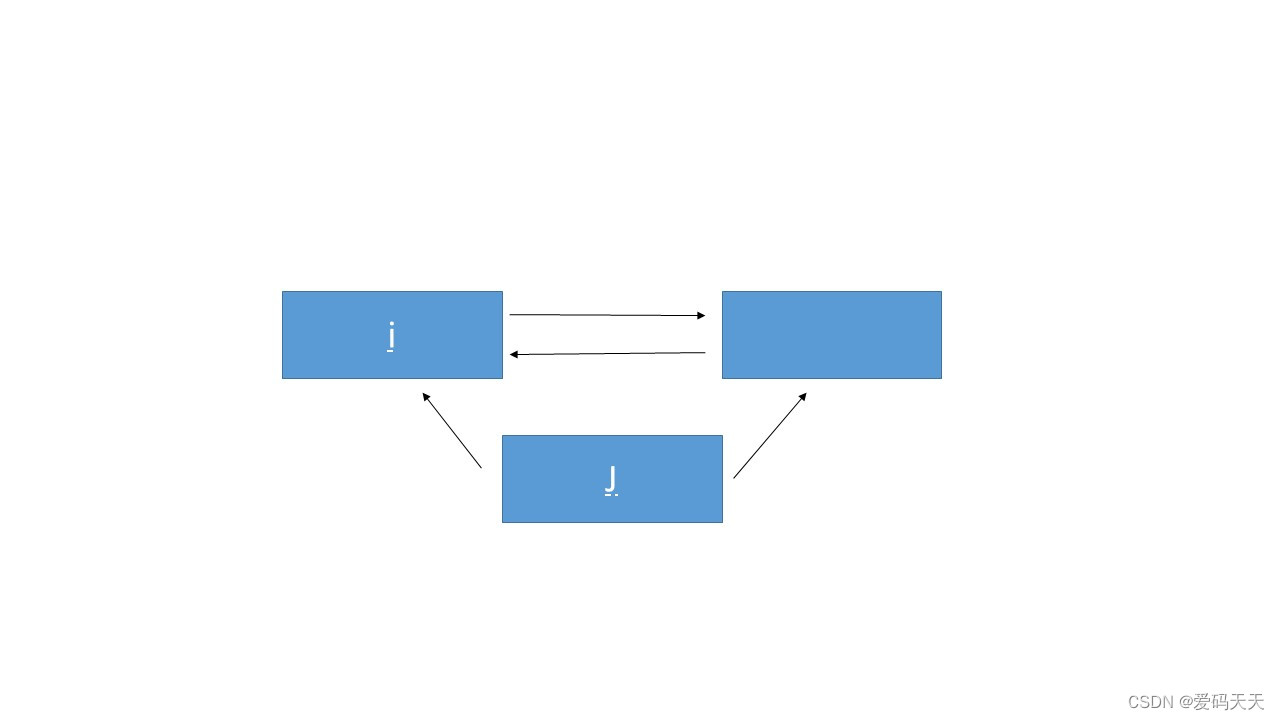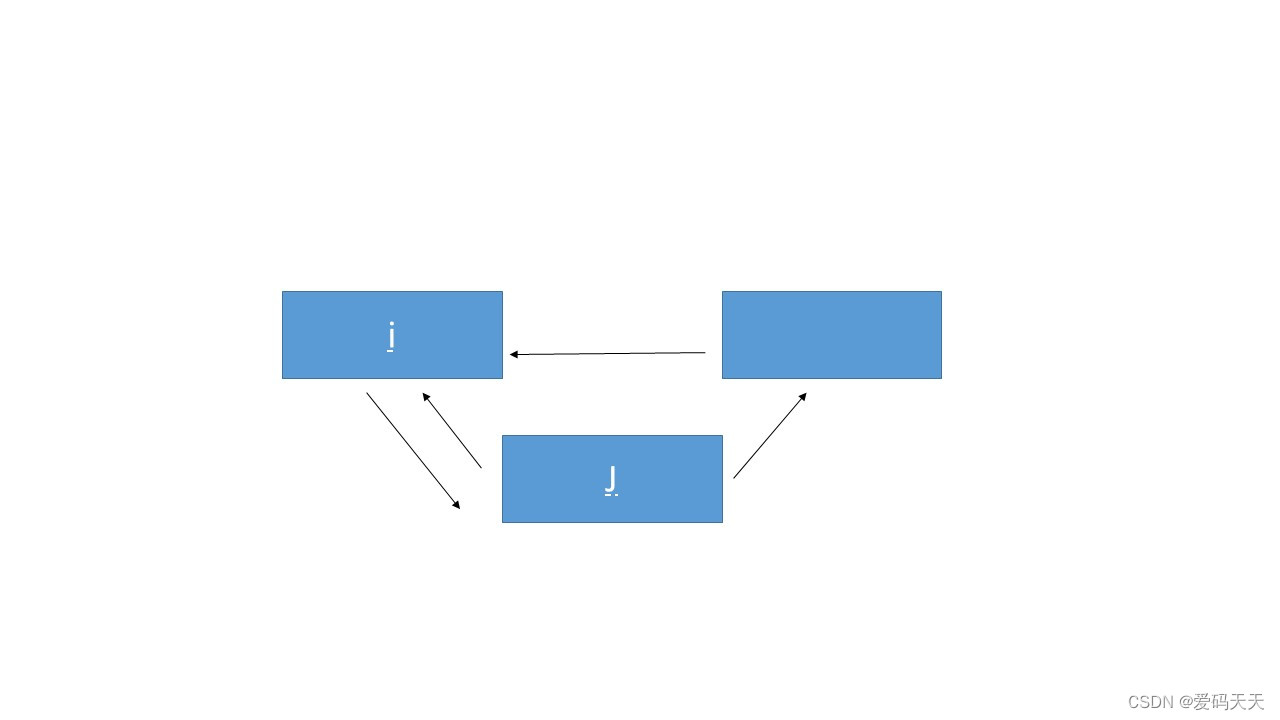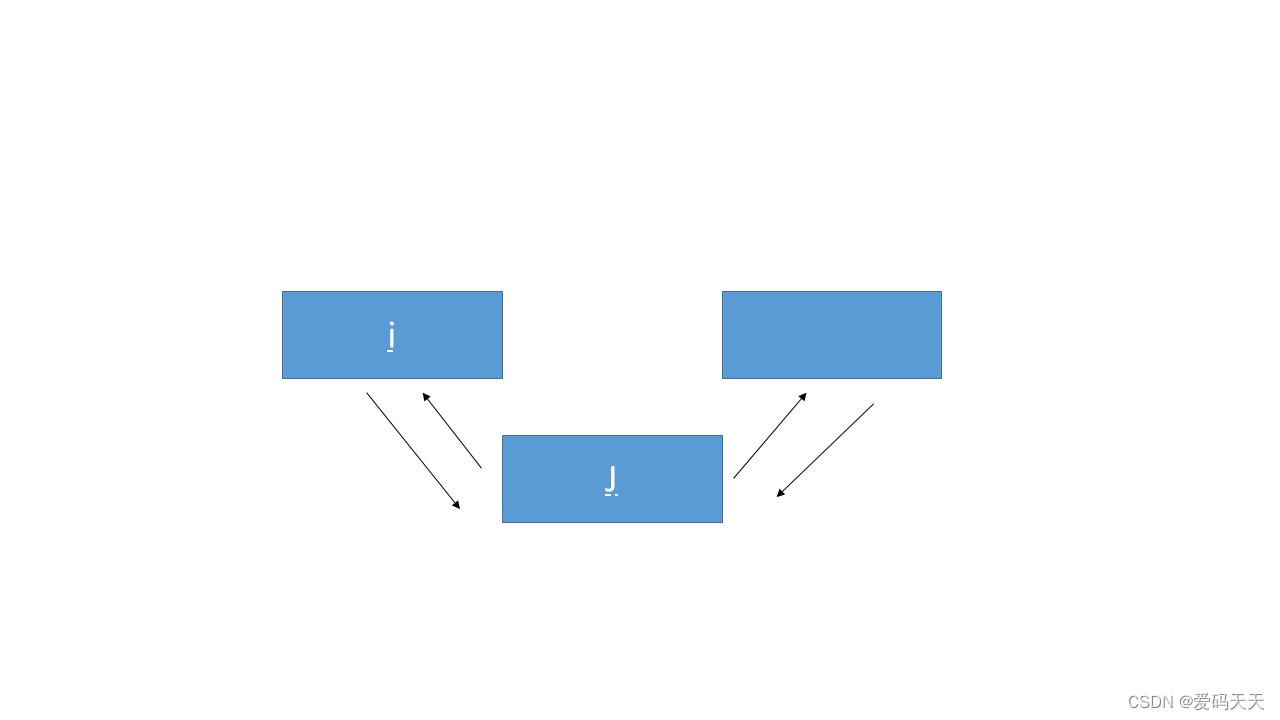完整代码如下：

p[j].right=p[i].right;

p[j].left=i;

p[i].right=j;

p[p[j].right].left=j;

持续输入数值后，就可以构成一个双向线表了。

#### （4）去除元素：

q[i].right=q[j].right;

q[q[j].right].left=i;

//将j的左右两元素连接


### 3.优缺点：

#### （2）缺点：

A.如果我们要插入或者删除的元素是在第一个位置，那么无疑的，我们需要移动大量的元素来完成这样的操作，而且限于线性表长度必须小于数组长度，如果我们需要插入大量数据，那么很难保证空间是否充足，而如果我们要删除大量数据的时候，无疑又会造成空间的浪费

B.顺序存储空间容易满，出现上溢，程序访问容易出问题，顺序存储结构下，存储空间不便扩充；

C.顺序存储空间的分配问题，分多了浪费，分少了空间不足上溢。

### 4.例题：

看一道十分典型的线性表，这道题也是最基础的类型。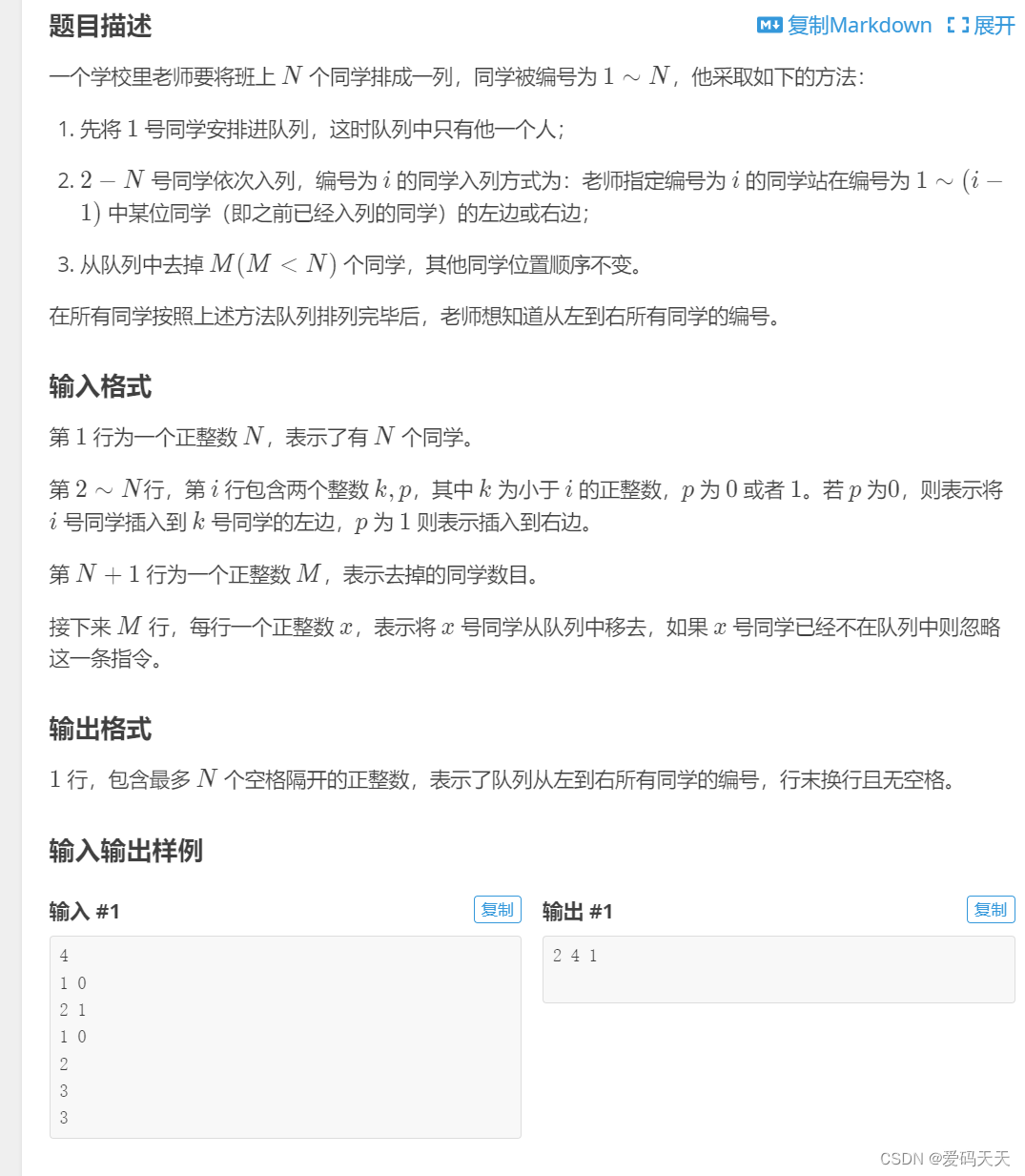#include<iostream>
using namespace std;

struct per{
int left;
int right;
int here;   //用于记录是否输出
} p;

int main(){
for(int i=0;i<100005;i++){
p[i].here=1;
}
int m,n;
int place,v;
p.left=0;
p.right=0;

//初始化0

p.left=0;
p.right=p.right;
p.right=1;
p[p.right].left=1;

//先将1号放在0右边

cin>>m;
for(int i=2;i<=m;i++){
cin>>v;
cin>>place;
if(place==1){
p[i].left=v;
p[i].right=p[v].right;
p[v].right=i;
p[p[i].right].left=i;
}

//向右插入

else{
p[i].left=p[v].left;
p[i].right=v;
p[v].left=i;
p[p[i].left].right=i;
}

//向左插入

}
cin>>n;
for(int j=0;j<n;j++){
int x;
cin>>x;
p[x].here=0;
}

//标记是否输出

for(int i=p.right;i!=0;i=p[i].right){
if(p[i].here==1&&p[i].right!=0) cout<<i<<" ";
else if(p[i].here==1&&p[i].right==0) cout<<i;
}

//从0开始向右输出

return 0;
}

这道题除去某元素时注意一下技巧，只用here标记一下是否在队列中。

## 二.map函数：

### 1.map的简介：

map的实质其实是映射，也就像数组，在定义数组时（如int array），其实是定义了一个从int型到int型的映射比如array=25、array=36就分别是将0映射到25、将4映射到36。         而一个double型数组则是将int型映射到double型，如db=3.14,double=0.01；

在数据储存上，map可以将任何基本类型（包括STL容器）映射到任何基本类型（包括STL容器），就比如map和vector , pair , map，set等镶嵌。但是如果是字符串到整型的映射，必须是string而不是char数组

### 2.map的基本用法：

#### （1）map的定义：

map的尖括号中包含两个元素，第一个是key值，另一个是value值。通过两者之间关系可以后续进行查找与赋值。

#### （2）map的赋值：

a.使用insert赋值：

//			(1).运 用 pair 函 数:

p.insert(pair<int,string>(1,"h1"));

//		    (2).再 次 运 用 map:

p.insert(map<int,string>::value_type(2,"h2"));     /*注意加上::value_type*/

//          (3).使用make_pair:

p.insert(make_pair(3,"h3"));

b.通过key赋value值：

	p="h0"; 

#### （3）判断是否插入成功：

    pair<map<int,string>::iterator,bool> insert_pair;
insert_pair=person.insert(map<int,string>::value_type(3,"lewis3"));

cout<<"是否插入成功："<<boolalpha<<insert_pair.second<<endl;

//boolalpha可以将bool类型的1和0转化为true与false 

#### （4）erase操作：

a.设置迭代器，将迭代器擦去；（命名迭代器方法为：map<类型1，类型2>::iterator）

迭代器定义可能比较陌生，记住格式即可。

b.直接擦key值

c.设置迭代器，设置范围，一起擦掉；

// 擦 除 步 骤
#include <iostream>
#include <map>
using namespace std;

int main ()
{
map<char,int> mymap;
map<char,int>::iterator it;
//插 入 元 素：
mymap['a']=10;
mymap['b']=20;
mymap['c']=30;
mymap['d']=40;
mymap['e']=50;
mymap['f']=60;

it=mymap.find('b');
mymap.erase (it);                   // 擦掉迭代器那一位

mymap.erase ('c');                  // 通过key值擦去

it=mymap.find ('e');
mymap.erase ( it, mymap.end() );    // 擦掉连续几个

// show content:
for (it=mymap.begin(); it!=mymap.end(); ++it)
cout << it->first << " => " << it->second << '\n';

return 0;
}

结果输出：

a => 10

d => 40

#### （5）find操作：

反之，map.find(key)=map.end();

map<char,int> my;

my['a']=50;
my['b']=100;
my['c']=150;
my['d']=200;

cout << "elements in mymap:" << '\n';
cout << "a => " << mymap.find('a')->second << '\n';
cout << "c => " << mymap.find('c')->second << '\n';
cout << "d => " << mymap.find('d')->second << '\n';


a => 50

c => 150

d => 200

#### （6）clear操作：

eg:设map变量为p:

p.clear();

#### （8）读取单个value：

eg：

map<int,string> person;

person="lewis0";

cout<<person;

#### （9）遍历读取map中元素：

输一个完整代码：

#include<iostream>
#include<map>
using namespace std;

int main()
{
map<int,string> person;

//一.赋 值 操 作：
//		1.直 接 赋 值：
person="lewis0";                                      /*每个赋值都要注意带上标号*/
person="lewis10";

//如 果 直 接 改 变 value，那 person 的 值 就 会 随 之 改 变

//		2.使 用 insert 赋 值：
//			(1).运 用 pair 函 数:
person.insert(pair<int,string>(1,"lewis1"));
//		    (2).再 次 运 用 map:
person.insert(map<int,string>::value_type(2,"lewis2"));   /*注意加上::value_type*/

//二.读 取 操 作：
//		1.一 般 法 读 取:
map<int,string>::iterator it;                  /*注意加上::iterator*/
for(it=person.begin();it!=person.end();it++)
{
cout<<it->first<<":"<<it->second<<endl;
}

//		2.使 用 auto 自 动 定 义 类 型：
for(auto it=person.begin();it!=person.end();it++)   /*auto有自动定义数据类型的功能，不必再定义it */
{
cout<<it->first<<":"<<it->second<<endl;
}

//  	3.使 用 auto 直 接 遍 历：
for(auto it:person)
{
cout<<it.first<<":"<<it.second<<endl;
}

//三.判 断 是 否 数 据 插 入 成 功:
pair<map<int,string>::iterator,bool> insert_pair;
insert_pair=person.insert(map<int,string>::value_type(3,"lewis3"));
cout<<"是否插入成功："<<boolalpha<<insert_pair.second<<endl;   //boolalpha可以将bool类型的1和0转化为true与false

//四.获取map中的元素:
map<int,string>::iterator it1;
it1=person.find(1);
if(it1!=person.end())
{
cout<<it1->second<<endl;
}
else
{
cout<<"not find"<<endl;
}

//五.删除的方法:
//		1.通过设置it:
map<int,string>::iterator it2;
it2 =person.find(1);
person.erase(it2);

//		2.通过直接改变key值:
person.erase(2);
for(auto it:person)
{
cout<<"-------------------------"<<endl;
cout<<it.first<<":"<<it.second<<endl;
}

//六.对字符进行操作：
map<string,string>person2;
person2["0"]="10";
person2["1"]="11";
person2["2"]="12";         ///只需要将数字改为字符

map<string,string>::iterator it3;
it3=person2.find("1");
person2.erase(it3);

for(auto it:person2)
{
cout<<it.first<<":"<<it.second<<endl;
}
cout<<endl<<endl;
cout<<person2["0"];
} 

#### （10）map与其他STL嵌套：

    map<int,vector<int> >Q;

for(int i=5;i<=10;i++){
Q.push_back(i);
}//向1000对应的vector里插入5 6 7 8 9 10

Q.erase(Q.begin(),Q.begin()+3);//删除1000对应的vector里前三个元素
for(int i=0;i<Q.size();i++){
cout<<Q[i]<<" ";
}
return 0;
}



其次，嵌套map，pair，具体应用参考例题。

### 3.例题：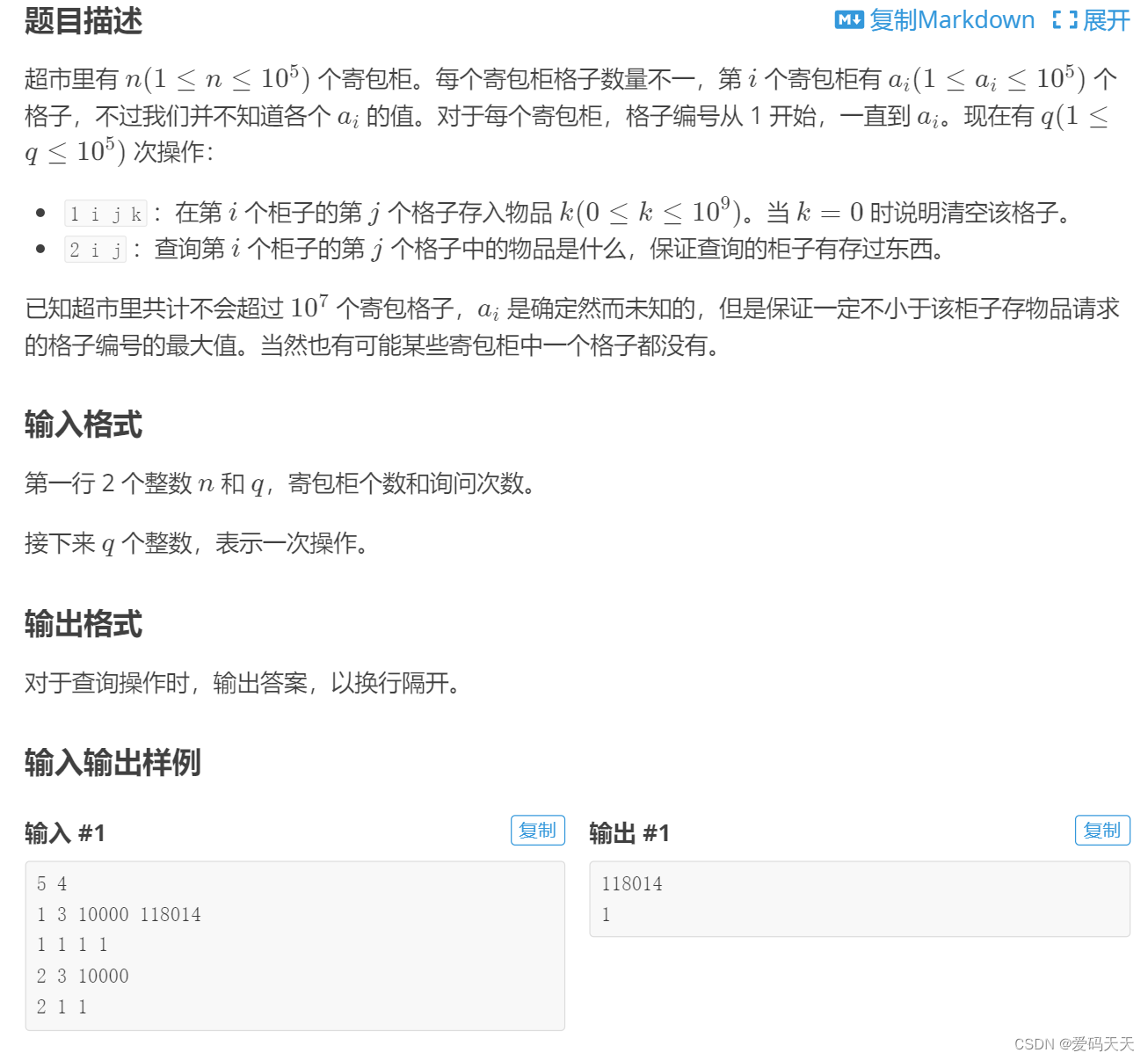#include<cstdio>
#include<iostream>
#include<map>//引用STL里的map库
using namespace std;
map<int,map<int,int> > a;//建立二维映射
int main(){
int n,q,x,y,k,z;
cin>>n>>q;//输入寄包柜个数及询问次数
for(int i=1;i<=q;++i){//循环q次
cin>>k>>x>>y;
if(k==1){//存放操作
cin>>z;
a[x][y]=z;//建立一次映射
}
else{//查询操作
cout<<a[x][y]<<endl;//直接输出所映射的值
}
}
return 0;
} 

#include <bits/stdc++.h>
using namespace std;
map <int, int> mat;  //二维map
int main() {
int n, q;
scanf("%d%d", &n, &q);//输入
while (q--) {
int x, i, j, k;
scanf("%d", &x);
if (x == 1) {
scanf("%d%d%d", &i, &j, &k);
mat[i][j] = k;  //直接映射
} else {
scanf("%d%d", &i, &j);
printf("%d\n", mat[i][j]); //直接查找对应元素，输出
}
}
return 0;
}

#include<cstdio>
#include<map>
using namespace std;
int n,q,p,k;
map<long long,int>b;
long long i,j;
int main()
{
scanf("%d%d",&n,&q);
while(q--)
{
scanf("%d%d%d",&p,&i,&j);
if(p==1)
{
scanf("%d",&k);
b[i*100000+j]=k;
}
else printf("%d\n",b[i*100000+j]);
}
return 0;
}

## 三.vector函数：

### 1.vector简介：

vector<int> a;           //无参数 - 构造一个空的vector,

vector<int> a(10);       //定义了10个整型元素的向量（尖括号中为元素类型名，它可以是任何合法的数据类型），但没有给出初值，其值是不确定的。

vector<int> a(10,1);     //定义了10个整型元素的向量,且给出每个元素的初值为1

vector<int> a(b);        //用b向量来创建a向量，整体复制性赋值， 拷贝构造

vector<int> a(b.begin(),b.begin+3);   //定义了a值为b中第0个到第2个（共3个）元素

int b={1,2,3,4,5,9,8};
vector<int> a(b,b+6);    //从数组中获得初值，b~b


### 2.vector用法：

#### （1）开头，结尾表示

a.front();

//返回第一个元素

a.back();

//返回最后一个元素

#### 将vector中元素清空，a.size()=0

a.clear();

//将vector中元素清空，a.size()=0

#### （3）检验是否为空

a.empty();

//判断是否空

#### （4） 删去最后一个元素

a.pop_back();

//即删去最后加入的元素

#### （5）删除操作

a.erase(a.begin()+1, a.begin()+3);
//删除a中第1个（从第0个算起）到第2个元素，也就是说删除的元素从a.begin()+1算起（包括它）一直到a.begin()+3（不包括它）


删除a中第1个（从第0个算起）到第2个元素，也就是说删除的元素从a.begin()+1算起（包括它）一直到a.begin()+3（不包括它）

#### （6）中间插入操作

a.加一个元素

a.insert(a.begin()+1,5);
//在第0位后1位上加入5  

b.加一串元素

a.insert(a.begin()+1, 3,5);
//在a的第1个元素（从第0个算起）的位置插入3个数，其值都为5


#### （7）尾部插入操作

a.push_back(k);
//在a的最后一个向量后插入一个元素，其值为k

#### （8）元素个数

a.size();
//数字个数

#### （9）最大容量

a.capacity()
//总容量大小

#### （10）调节元素个数

a.不赋值改变个数，如果需要补位，则出随机值；

如果位数过多，删除多余项

a.resize(10);
//多删少补

b.将补位元素赋值：

a.resize(10,2)
//如果数位不足，补2

#### （11）调节容量大小

a.reserve(100)
//将容量调节至100

08-30
07-05407
07-24919
01-18221
10-09236
08-131603
05-291910
07-262029
08-071829
08-29
09-061012
04-201万+
12-03850
03-18258
10-17118

### “相关推荐”对你有帮助么？

•非常没帮助
•没帮助
•一般
•有帮助
•非常有帮助被折叠的  条评论 为什么被折叠?到【灌水乐园】发言¥1 ¥2 ¥4 ¥6 ¥10 ¥20获取中扫码支付点击重新获取扫码支付1.余额是钱包充值的虚拟货币，按照1:1的比例进行支付金额的抵扣。
2.余额无法直接购买下载，可以购买VIP、付费专栏及课程。余额充值